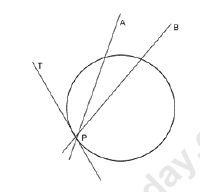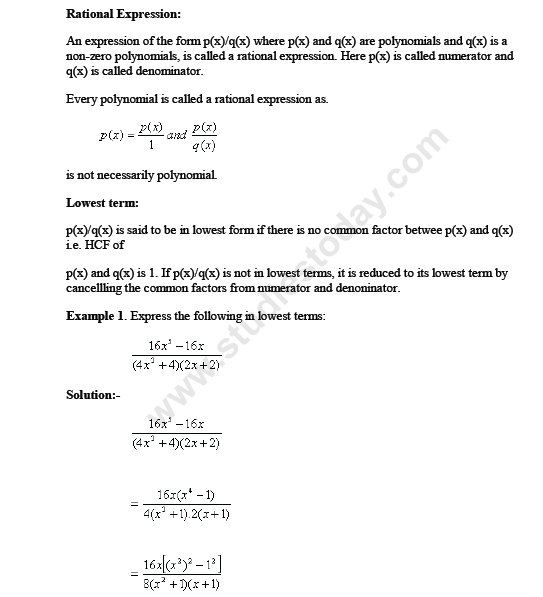# CBSE Class 10 Mathematics Books

• ### CBSE Class 10 Maths Trigonometry

Please refer to attached file for CBSE Class 10 Maths Trigonometry

• ### CBSE Class 10 Maths Tangents to a circle

Tangent to a circle is a line which intersects the circle in exactly one point. At a point of a circle there is one and only one tangent. The tangent at any point of a circle is perpendicular to the radius through the point of contact. The lengths of tangents drawn from an external point to a...

• ### CBSE Class 10 Maths Statistics

Chapter 15 Statistics Statistics Statistics is the science which studies the methods and techniques used in the collection, presentation and analysis of data and draws. conclusion on the basis of the same. The statistics is thus, used in two senses: 1. In the sense of data and 2. In the sence of...

• ### CBSE Class 10 Maths Similar Triangles

Chapter 8 Similar Triangles Similar Triangles: Whenever we talk about two congruent figures then they have the ‘same shape’ and the ‘same size’. There are figures that are of the ‘same shape but not necessarily of the ‘same size’. They are said to be similar. Congruent figures are similar but...

• ### CBSE Class 10 Maths Rational Expression

Please refer to attached file for CBSE Class 10 Maths Rational Expression

# CBSE Class 10 Maths TrigonometryPlease refer to attached file for CBSE Class 10 Maths Trigonometry

Click for more Trigonometry Study Material
 CBSE Class 10 Maths Height and Distance CBSE Class 10 Maths Trigonometry NCERT Class 10 Maths Introduction To Trigonometry NCERT Class 10 Maths Introduction To Trigonometry Questions NCERT Class 10 Maths Some Applications Of Trigonometry

# CBSE Class 10 Maths Tangents to a circle

Tangent to a circle is a line which intersects the circle in exactly one point. At a point of a circle there is one and only one tangent.Click for more Mathematics Study Material
 CBSE Class 10 Maths Geometrical Construction CBSE Class 10 Maths HCF and LCM CBSE Class 10 Maths Income Tax CBSE Class 10 Maths Instalment CBSE Class 10 Maths Rational Expression CBSE Class 10 Maths Tangents to a circle NCERT Class 10 Maths Answers and Solutions NCERT Class 10 Maths Constructions NCERT Class 10 Maths Constructions Questions NCERT Class 10 Maths Design of Question Paper and Answers1 NCERT Class 10 Maths Design of Question Paper and Answers2 NCERT Class 10 Maths Mathematical Modelling NCERT Class 10 Maths Proofs In Mathematics

# CBSE Class 10 Maths Rational ExpressionPlease refer to attached file for CBSE Class 10 Maths Rational Expression

Click for more Mathematics Study Material
 CBSE Class 10 Maths Geometrical Construction CBSE Class 10 Maths HCF and LCM CBSE Class 10 Maths Income Tax CBSE Class 10 Maths Instalment CBSE Class 10 Maths Rational Expression CBSE Class 10 Maths Tangents to a circle NCERT Class 10 Maths Answers and Solutions NCERT Class 10 Maths Constructions NCERT Class 10 Maths Constructions Questions NCERT Class 10 Maths Design of Question Paper and Answers1 NCERT Class 10 Maths Design of Question Paper and Answers2 NCERT Class 10 Maths Mathematical Modelling NCERT Class 10 Maths Proofs In Mathematics

# CBSE Class 10 Maths Instalment

Chapter 6

Instalment

Instalments

Click for more Mathematics Study Material
 CBSE Class 10 Maths Geometrical Construction CBSE Class 10 Maths HCF and LCM CBSE Class 10 Maths Income Tax CBSE Class 10 Maths Instalment CBSE Class 10 Maths Rational Expression CBSE Class 10 Maths Tangents to a circle NCERT Class 10 Maths Answers and Solutions NCERT Class 10 Maths Constructions NCERT Class 10 Maths Constructions Questions NCERT Class 10 Maths Design of Question Paper and Answers1 NCERT Class 10 Maths Design of Question Paper and Answers2 NCERT Class 10 Maths Mathematical Modelling NCERT Class 10 Maths Proofs In Mathematics

## Latest NCERT & CBSE News

Read the latest news and announcements from NCERT and CBSE below. Important updates relating to your studies which will help you to keep yourself updated with latest happenings in school level education. Keep yourself updated with all latest news and also read articles from teachers which will help you to improve your studies, increase motivation level and promote faster learning

### CBSE Board Examination Scheme of Assessment 2021 2022

A. Academic session to be divided into 2 Terms with approximately 50% syllabus in each term: The syllabus for the Academic session 2021-22 will be divided into 2 terms by following a systematic approach by looking into the interconnectivity of concepts and topics by...

This has a reference to the letter no CBSE/COORD/2021 dated 11.02.2021 regarding conduct of the School-Based Assessment (Internal Assessment/Practical/ Project) of Class-XII and uploading the marks by 11.06.2021. It has been observed that some schools have not been...

### CBSE Class 12 Evaluation Criteria 2021

SUMMARY OF THE POLICY FOR TABULATION OF MARKS FOR CLASS XII BOARD EXAMINATIONS 2021   1(a) Due to cancellation of the Board examinations, the assessment of theory portion of 80/70/60/50/30 marks will be done by the school based on the following:     Class...

### Moderation of Marks Class 11 and 12 Board Exams

The portal for moderation and finalization of results for Class-12 is being opened from 16.07.2021 to 22.07.2021. As Board has to declare the result latest by 31.07.2021, schools have been requested to follow the schedule strictly and complete the moderation within...

### FAQs on Policy for Tabulation of Marks for Class 10 Board Exams

ON POLICY Question.1 How CBSE will declare the result of Class-X? Answer. Results of Class X Board will be declared on the basis of an objective criterion developed by the Board vide Notification no.CBSE/CE/2021 dated 01.05.2021. Question.2. If any candidate is not...

×